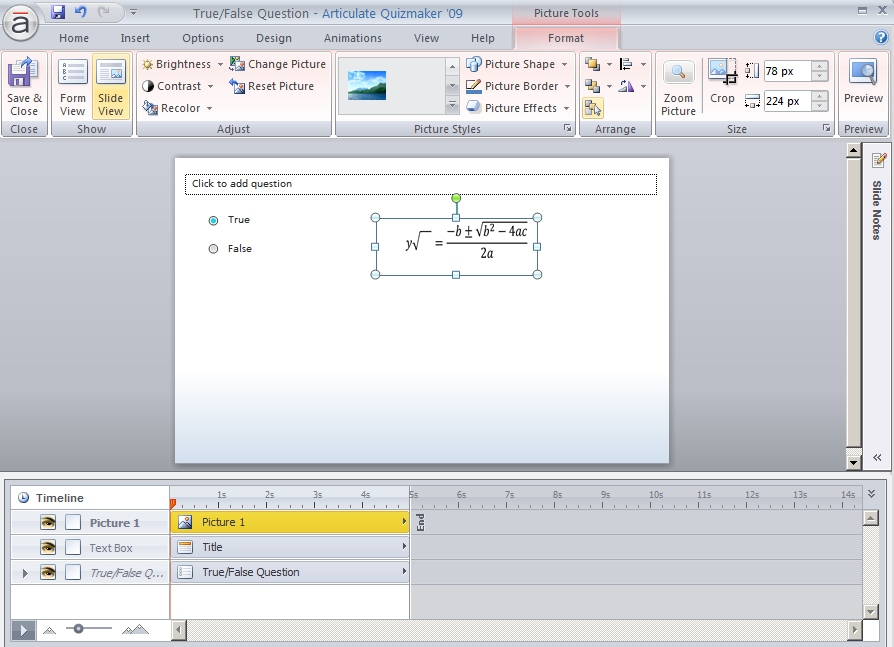# Need math equation in the questions and options

Is it possible to give math equation in Articulate Quizmaker insert Math equation in between the questions and also in the options for eg: x + 13  or cube root of x + 13.

3

###### 7 RepliesYes, I think you can do that. There's an "equation editor" in Word. You can create the equation there, then copy and paste it as an image into Quizmaker.

Here's en example:And here's an artcle that explains the process:

http://office.microsoft.com/en-us/word-help/write-insert-or-change-an-equation-HA001230361.aspx?CTT=5&origin=HA001230366#_Toc280198104

I hope that helps!